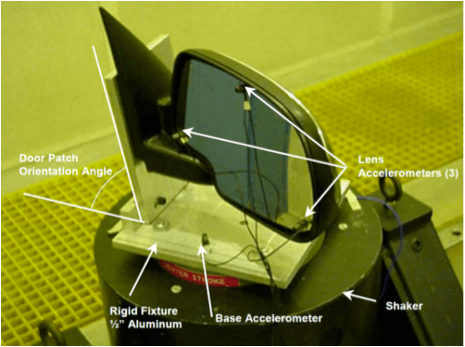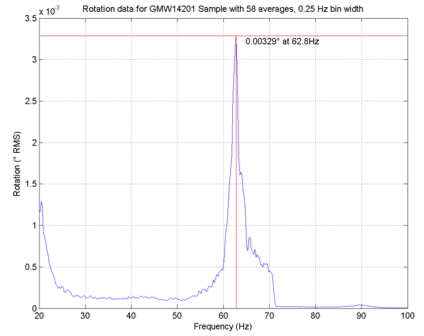# VN0011 - Mirror Vibration Evaluation Procedure

## AbstractThe GMW14201 specification (Mirror Vibration Evaluation Procedure) is described.

### QUESTION

Many people have asked what the GMW14201 specification is and what is needed to perform a test that matches this specification.

The GMW14201 specification is a rotational vibration measurement test implemented on inside and outside automotive rear view mirrors. It is designed to measure the rotational response of a mirror when subjected to vertical input vibration.

TEST SETUP METHOD

Accelerometers are placed on the reflective surface of an inside or outside rearview mirror in a triangular pattern. This triangular pattern is highlighted in red in Figure 1 below.The distance between each of the accelerometers is entered into a form in VibrationVIEW for later calculations. The mirror assembly is then mounted to a rigid fixture which is mounted to the head of a shaker. The mirror is then shaken vertically by using a random vibration profile specified by the GMW14201 specification. While the test is conducted, VibrationVIEW records the values of each of the input and response accelerometers at a high sample rate. Note: recording waveform data requires the RecorderVIEW option on the VR controller conducting the test.

POST PROCESSING METHOD

After the test has completed, a MATLAB script computes the remaining calculations. The accelerometers are arranged in a way that the difference in acceleration between two accelerometers divided by the distance between them determines the angular acceleration between them. Figure 2, below, is a 2-D representation of the triangular array shown mounted on a mirror in Figure 1.X1, X2, and X3 in Figure 2 represent the readings of the three physical accelerometers shown in Figure 1. X4 is a “virtual”, calculated accelerometer. A13 and A24, shown in Figure 2, denote the angular acceleration that is calculated between points 1 and 3, and 2 and 4 respectively. A13 represents the “yaw” of the mirror, while A24 represents the “pitch” of the mirror.

### TEST RESULTS

The MATLAB script mentioned before outputs a series of graphs. One is “Transmissibility vs. Frequency”:And another is “Rotation vs. Frequency”:### TOOLS REQUIRED

The GMW14201 specification can be conducted with any capable hardware and software combination by following the details of the specification. However, it is much easier to carry out the test by using VR’s hardware, software, and custom MATLAB script. The following list includes the recommended tools for conducting the test:

1. GMW14201 Specification from General Motors Corporation
2. VR9500 or VR8500 Vibration Controller
3. RandomVIEW Test Module
4. RecorderVIEW waveform recording option
5. GMW14201 MATLAB script